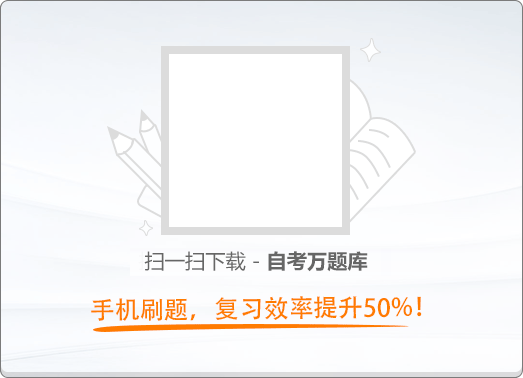150:002015年4月《大学语文》真题

1
(单项选择题)

• A.

五亩之宅，树之以桑

• B.

鸡豚狗彘之畜，无失其时

• C.

百亩之田，勿夺其时

• D.

谨庠序之教，申之以孝悌之义

• A
• B
• C
• D

2
(单项选择题)

《咬文嚼字》主要采用的论证方法是（　）。

• A.

类比法

• B.

对比法

• C.

归纳法

• D.

演绎法

• A
• B
• C
• D

3
(单项选择题)

《吃饭》一文提及的古代典籍中，把“做宰相”比为“和羹调鼎”的是（　）。

• A.

《尚书》

• B.

《礼记》

• C.

《论语》

• D.

《吕氏春秋》

• A
• B
• C
• D

4
(单项选择题)

• A.

弹铗三歌

• B.

焚券于薛

• C.

西游于梁

• D.

立庙于薛

• A
• B
• C
• D

5
(单项选择题)

《种树郭橐驼传》的写作目的是（　）。

• A.

称赞长人者的勤勉

• B.

讽刺长安富豪的奢侈

• C.

批评执政者的繁苛

• D.

阐述正确的种树方法

• A
• B
• C
• D

6
(单项选择题)

《马伶传》中，侯方域所讥讽的当朝权奸、被马伶称为“昆山相国”的是（　）。

• A.

阮大铖

• B.

顾秉谦

• C.

严嵩

• D.

魏忠贤

• A
• B
• C
• D

7
(单项选择题)

《西湖七月半》“断桥石磴始凉”一句是表示（　）。

• A.

农历七月半已至秋凉季节

• B.

游客散尽，西湖冷清下来

• C.

杭人游湖，巳出酉归，此时已到傍晚

• D.

月光澄澈，给人一种寒冷的幻觉

• A
• B
• C
• D

8
(单项选择题)

• A.

带来光明的思想者

• B.

抒情主人公的高洁人格和精神

• C.

黑暗势力的一部分

• D.

遭受蹂躏却还抱有希望的弱者

• A
• B
• C
• D

9
(单项选择题)

《纪念傅雷》的作者最欣赏傅雷的一点是（　）。

• A.

艺术的涵养，知识学问的累积

• B.

性情刚直，如一团干柴烈火

• C.

望子成龙的心情如此之热烈

• D.

具有浩然之气的儒家之刚者

• A
• B
• C
• D

10
(单项选择题)

《诗经》中的《蒹葭》属于（　）。

• A.

秦地民歌

• B.

楚地民歌

• C.

京都乐歌

• D.

祭祀曲辞

• A
• B
• C
• D

11
(单项选择题)

• A.

问是谁家姝

• B.

罗敷年几何

• C.

何用识夫婿

• D.

宁可共载不

• A
• B
• C
• D

12
(单项选择题)

• A.

停杯投箸不能食

• B.

闲来垂钓碧溪上

• C.

忽复乘舟梦日边

• D.

长风破浪会有时

• A
• B
• C
• D

13
(单项选择题)

• A.

《秋兴八首》（其一）

• B.

《早雁》

• C.

《虞美人》（春花秋月何时了）

• D.

《声声慢》（寻寻觅觅）

• A
• B
• C
• D

14
(单项选择题)

• A.

是处红衰翠减，苒苒物华休

• B.

惟有长江水，无语东流

• C.

叹年来踪迹，何事苦淹留

• D.

争知我、倚栏干处，正恁凝愁

• A
• B
• C
• D

15
(单项选择题)

《鹧鸪天》（重过阊门万事非）中，“空床卧听南窗雨，谁复挑灯夜补衣”所用抒情方式是（　）。

• A.

借举止抒情

• B.

借叙事抒情

• C.

借比喻抒情

• D.

借典故抒情

• A
• B
• C
• D

16
(单项选择题)

《摸鱼儿》（更能消几番风雨）所写景物属于的时令是（　）。

• A.

初春

• B.

暮春

• C.

初秋

• D.

深秋

• A
• B
• C
• D

17
(单项选择题)

《一句话》中，“咱们的中国”指的是（　）。

• A.

人民当家作主的中国

• B.

历史悠久的中国

• C.

海外游子牵挂的中国

• D.

多灾多难的中国

• A
• B
• C
• D

18
(单项选择题)

• A.

一座荒林

• B.

一根常春藤

• C.

一块云朵

• D.

一所小草棚

• A
• B
• C
• D

19
(单项选择题)

《宝黛吵架》写黛玉爱之至深反生怨情的表现是（　）。

• A.

提起张道士说亲的话头

• B.

痛哭呕药

• C.

在潇湘馆临风洒泪

• D.

抢铰玉石穗子

• A
• B
• C
• D

20
(单项选择题)

• A.

《断魂枪》

• B.

《哦，香雪》

• C.

《金鲤鱼的百裥裙》

• D.

《苦恼》

• A
• B
• C
• D